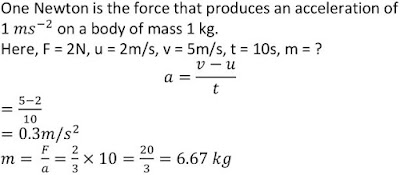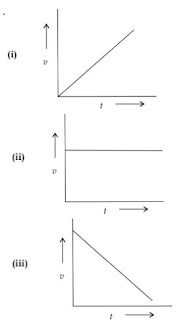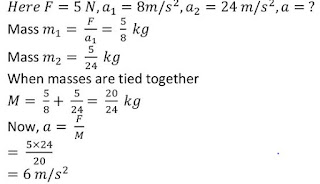## Extra Questions for Class 9th: Ch 9 Force and Laws of Motion (Science) Important Questions Answer Included

Very Short Answer Questions (VSAQs): 1 Mark

Q1. State the meaning of recoil velocity of a gun?
The velocity with which a gun moves backward after firing a bullet is called the recoil velocity of a gun.

Q2. Name the force which is responsible for change in position or state of an object.
Unbalanced forces.

Q3. Name the unbalanced force which slows down a moving bicycle when we stop pedaling it.
Force of friction.

Q4. Give one point of difference between balanced and unbalanced forces.
When forces acting on a body from all sides are equal, they cancel effect of each other and are known as balanced forces. On the other hand, when forces acting on a body are not equal/do not cancel each other are called unbalanced forces.

Q5. Why does the sole of the shoe wears out?
Sole of the shoe wears out due to friction between sole of the shoes and earth’s surface.

Q6. Mass of a body is doubled. How does its acceleration change under a given force?
Acceleration becomes half.

Q7. Mention any two kinds of changes that can be brought about in a body by force.
Change in speed/change of direction/change of shape.

Q8. State two effects, a force can produce in a non rigid body fixed at a position.
Change its shape and size.

Short Answer Questions-I (SAQs-I) : 2 Marks

Q1. State the SI unit of pressure. Mention the unit which we use to measure pressure exerted by a gas. What do you understand by normal atmospheric pressure?

Pascal Atmosphere (atm)
Atmospheric pressure at sea level = 1 atm

Q2. Define SI unit of force. A force of 2N acting on a body changes its velocity uniformly from 2 m/s to 5m/s in 10s. Calculate the mass of the body.Q3. (i) It is possible that the train in which you are sitting appears to move while it is at rest? Justify your answer.
(ii) Can the train appear to be at rest while moving? Justify your answer.

(i) Yes, this is because of relative motion.
(ii) Yes. This is because of inertia. We are in state of motion when compared to the train so we feel that the train is moving when actually it is at rest.

Q4. While driving vehicle how the use of safety belts prevents accidents? To show that a body remains at rest unless acted upon by an unbalanced force, mention one situation from everyday life.

First law of motion-makes the forward motion slower during accidents.
Example: Pedaling of bicycle.
When we stop pedaling, the bicycle begins to slow down. This is again because of the friction forces acting opposite to the direction of motion. In order to keep the bicycle moving, we have to start pedaling again. It thus appears that an object maintains its motion under the continuous application of an unbalanced force.

Q5. Derive Newton’s first law of motion from the mathematical expression of the second law of motion.

Newton’s first law states that a body stays at rest if it is at rest and moves with a constant velocity unit if a net force is applied on it. Newton’s second law states that the net force applied on the body is equal to the rate of change in its momentum.
F= ma
F = m(v-u)/t
Ft = mv-mu
That is, when F = 0, v = u for whatever time, t is taken. This means that the object will continue moving with uniform velocity, u throughout the time, t. If u is zero than v will also be zero, i.e., object will remain at rest.

Q6. Why is it easier to stop a tennis ball in comparison to a cricket ball moving with the same speed?

Tennis ball is lighter (less mass) than a cricket ball. Tennis ball moving with same speed has less momentum (mass × velocity) than a cricket ball. It is easier to stop tennis ball having less momentum.

Short Answer Questions-II (SAQs-II) : 3 Marks

Q1.What type of force is acting in the cases given above ?

(i) Accelerating unbalanced force.
(ii) No force.
(iii) Retarding unbalanced force.

Q2. A force of 5N produces an acceleration of 8 m/s2 in mass m1, and acceleration of 24 m/s2 is mass m2. What acceleration would be if both are tied together?Q3. Two boys dropped sheets of paper from the top of the tower. One boy dropped his sheet of paper as such, while the other boy dropped it by changing it in the shape of a ball. Which sheet of paper will reach the ground first? Explain why? (Assume air-resistance negligible).

The sheet shaped in form of a ball falls before the open sheet. Because the open sheet experiences more air resistance due to its large surface-area and this restricts its fall.

Q4. What are the effects of the following on inertia of a body?
(i) If force is doubled
(ii) If density is halved
(iii) If volume is reduced to one third.

(i) No effect.
(ii) No effect.
(iii) No effect.

Q5. What type of motion is represented by each of the following graphs?(i) Uniform motion.
(ii) Uniform accelerated motion.
(iii) Uniform motion.

Long Answer Questions (LAQs) : 5 Marks

Q1. What is meant by ‘inertia’? What are different types of inertia? Give two examples in each case.

Inability of the body to change by itself its state of rest or state of uniform motion is called inertia.
Types: Inertia of rest: e.g. :
(i) When a card is flicked with a finger the coin placed over it falls in the tumbler.
(ii) Only the carom coin at the bottom of a pile is removed when a fast moving carom striker hits it.
Inertia of motion: e.g. :
(i) When a moving bus stops suddenly, the luggage might slide towards the front side of the bus and fall.
(ii) We tend to fall forward when a bus suddenly stops.

Q2. (i) Define momentum. Write its S.I. unit.
(ii) How much momentum will an object of mass 10 kg transfer to the floor, if it falls from a height of 5 m (g =10 m/s2).
(iii) Explain how a karate player can break a pile of tiles with a single blow of his hand.

(i) Momentum is the product of mass and velocity.
SI unit of momentum is – kg m/s.
(ii) v2=u2+2gh
v2= (0)2+2(10)(5)
v2=100
∴v=10 m/s

(ii) Momentum=m×v
=10×10=100 kg m/s

(iii) The karate player strikes the pile of tiles with his hand very fast. In doing so, the large momentum of fast moving hand is reduced to zero in a very short time. This exerts a very large force on the pile of tiles which is sufficient to break them.

Q3. (a) State the law of conservation of momentum.
(b) Observe the following diagram and answer the questions given below:
(i) Which direction does the balloon move when the thread tied to its neck is removed and why?
(ii) State the conclusion drawn from this activity.

(a) When two or more bodies act upon one another their total momentum remains constant, provided no external forces are acting.

(b) (i) Air from inside the balloon escapes from the mouth of the balloon. Balloon moves in opposite direction that is from left to right.
(ii) Forces of action and reaction are equal and opposite.

Q4. (i) Two objects of masses 100 g and 200 g are moving along the same line and direction with velocities of 2 m/s and 1 m/s respectively. They collide and after the collision, the first object moves at a velocity of 1.67 m/s. Determine the velocity of second object.
(ii) If a man jumps out from a boat, the boat moves backwards. Why?

Let
(i) m1=100=0.1 kg
m2=200 g=0.2 kg
u1=2 m/s
u2=1m/s
v1=1.67 m/s

According to law of conservation of momentum
m1u1+m2u2= m1v1+ m2v2
0.1×2 + 0.2×1 = 0.1 ×1.67 + 0.2×v2
0.2 + 0.2 = 0.167 + 0.2 v2
v2 =1.165 m/s

(ii) It is based on Newton’s third law of motion. As boat is floating and is not fixed, so it moves backwards.